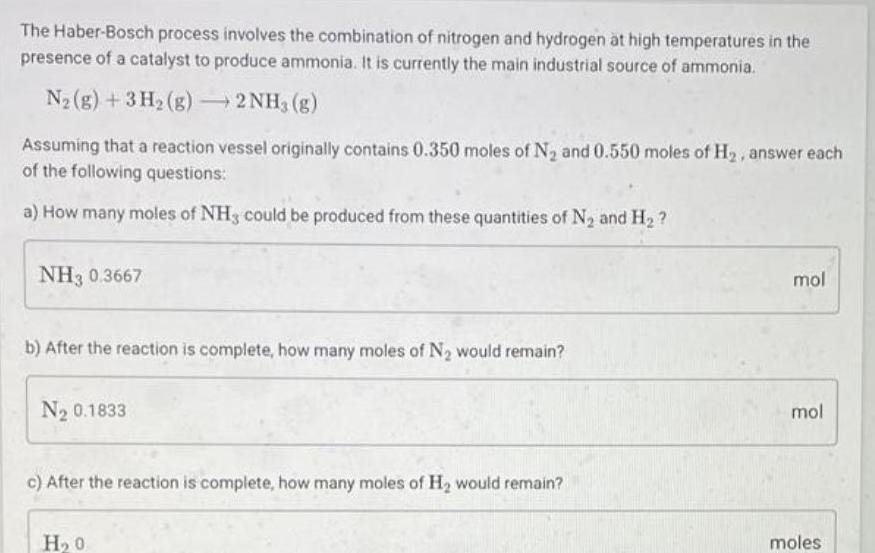Question:

# The Haber Bosch process involves the combination of nitrogen

Last updated: 6/28/2023The Haber Bosch process involves the combination of nitrogen and hydrogen at high temperatures in the presence of a catalyst to produce ammonia It is currently the main industrial source of ammonia N g 3H g 2NH3 g Assuming that a reaction vessel originally contains 0 350 moles of N and 0 550 moles of H answer each of the following questions a How many moles of NH3 could be produced from these quantities of N and H NH3 0 3667 b After the reaction is complete how many moles of N would remain N 0 1833 c After the reaction is complete how many moles of H would remain H 0 mol mol moles